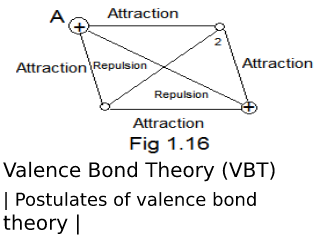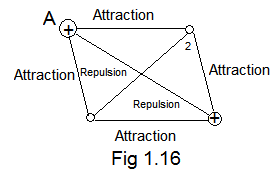# Valence Bond Theory (VBT) | Postulates of valence bond theory |

##Heitler and F. London calculated theoretically the bond energy and bond length of H with the help of wave mechanics. This treatment became known as the valence bond theory (VBT). Later on, it was modified by L. Pauling and J.E. Slater.

Consider the formation of H2 from two hydrogen atoms HA  and HB. Their wave functions are ψA (1) ψB (2) respectively. The numbers 1 and 2 represent their electron.
In order to understand the formation of the bond between two H-atom we will discuss the following two situations:
1. When two atoms are far apart.
2. When two atoms are close enough to form a bond. Their intermolecular distance is equal to that of bond lengths.

### First Situation:

When the two atoms are far apart, there is no interaction between the electrons and the atoms. The wave function for such a system is,

φmo     =       ψA (1) . ψB (2)      ………   (i)

When this equation is solved for energy and bond length, it is observed that the total energy of the system is equal to the sum of energies of individual atoms. It means that no bond is formed because in the formation of a bond the energy of the system must be less than the energy of combining atoms.

### Second Situation:When two atoms are brought closer so that their internuclear distance is equal to the bond length. At such a close distance the interactions of nuclei and electrons have to be considered. Heitler and London pointed out that it is not possible to restrict the electrons to their own nuclei. One electron is as a good part of an atom as it is of the other atom and thus the restriction of the electron is not possible. They suggested an approximate wave function which allows both arrangements:

φmo     =       ψA (1) . ψB (2)  +  ψA (2) . ψB (1)The energy is calculated with (ii) is the minimum of value 303 KJ/mole for a bond length of 0.80 Aº. The lowering of energy is due to the fact that electron is attracted by both nuclei and thus they have a greater volume to move about.

Can be a further improvement can be made in wave function (ii) by considering the possibility that both electrons may occupy the same molecular orbital i.e., the electron 1 of H may occupy the orbital of an atom HB and vice versa. This is a situation in which the bond is ionic,

for this situation, the wave function of the H system will be

φionic     =       ψA (1) . ψA (2)  +  ψB (1) . ψB (2)          ……….. (iii)

If the energy is calculated taking into consideration the ionic bonding also, then the overall wave function will become:
φMO   =  φCOV  +  λφionic               ……    (iv)

Where λ = contribution of ionic character in H2 molecule. It is 103 KJ/mole. The bond length comes out to be 0.75ºA and the total energy of φMO is near to the actual value, 435 KJ/mole.

In this discussion, elections are supposed to obey Pauli’s exclusion Principle, i.e., the electron is paired in a molecular orbital. If they have the same spins, the wave function for the system will be some that different

φMO   =  ψA (1) . ψB (2)  +  ψA (1) . ψB (2)  ….. (v)

### Postulates of valence bond theory.

“Postulates of valence bond theory”

1. Only half fill orbitals take part in bond formation.
2. No of bonds formed by an atom is equal to its number of unpaired electrons.
3. A molecular orbital is formed by the linear overlap of atomic orbitals.
4. The pairing of electron results in bond energy.
5. The electron pair is localized between two nuclei.
6. The overlap is a measure of the bond strength. Greater the overlap, shorter and stronger will be bond length.
7. Resonance is permissible in VBT.
8. It also accounts for the ionic character of a covalent bond and the hybridization of atomic orbitals.
9. Co-ordination bonding is not explainable on the basis of this theory.